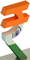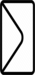# Transactional Proofs (Some Random Transactions)

PROOF_PDF01      PROOF_R0001      PROOF_R0002      PROOF_R0003      PROOF_R0004

PROOF_R0005      PROOF_R0006      PROOF_R0007      PROOF_R0008      PROOF_R0009

PROOF_R0010      PROOF_R0011      PROOF_R0012      PROOF_R0013      PROOF_R0014

PROOF_R0015      PROOF_R0016      PROOF_R0017      PROOF_R0018      PROOF_R0019

PROOF_R0020      PROOF_R0021      PROOF_R0022      PROOF_R0023      PROOF_B2335

PROOF_B2334      PROOF_S0837      PROOF_B2333      PROOF_B2332      PROOF_B2331

PROOF_S0836      PROOF_B2330      PROOF_B2329      PROOF_B2328      PROOF_S0835

PROOF_B2327      PROOF_B2326      PROOF_B2325      PROOF_B2324      PROOF_B2323

PROOF_B2322      PROOF_B2321      PROOF_B2320      PROOF_B2319      PROOF_B2318

PROOF_B2317      PROOF_S0834      PROOF_B2316      PROOF_B2315      PROOF_B2314

PROOF_S0833      PROOF_B2313      PROOF_B2312      PROOF_B2311      PROOF_S0832

PROOF_S0831      PROOF_B2310      PROOF_B2309      PROOF_B2308      PROOF_B2307

PROOF_B2306      PROOF_B2305      PROOF_S0830      PROOF_B2304      PROOF_S0829

PROOF_B2303      PROOF_B2302      PROOF_B2301      PROOF_B2300      PROOF_B2299

PROOF_B2298      PROOF_S0828      PROOF_B2297      PROOF_S0827      PROOF_B2296

PROOF_B2295      PROOF_B2294      PROOF_B2293      PROOF_B2292      PROOF_B2291

PROOF_B2290      PROOF_B2289      PROOF_B2288      PROOF_B2287      PROOF_B2286

PROOF_S0826      PROOF_B2285      PROOF_B2284      PROOF_B2283      PROOF_B2282

PROOF_B2281      PROOF_B2280      PROOF_B2279      PROOF_S0825      PROOF_B2278

PROOF_B2277      PROOF_B2276      PROOF_B2275      PROOF_S0824      PROOF_B2274

PROOF_B2273      PROOF_B2272      PROOF_B2271      PROOF_B2270      PROOF_B2269

PROOF_B2268      PROOF_B2267      PROOF_B2266      PROOF_S0823      PROOF_B2265

PROOF_S0822      PROOF_S0821      PROOF_B2264      PROOF_B2263      PROOF_B2262

PROOF_S0820      PROOF_S0819      PROOF_B2261      PROOF_B2260      PROOF_B2259

PROOF_S0818      PROOF_B2258      PROOF_B2257      PROOF_B2256      PROOF_B2255

PROOF_B2254      PROOF_B2253      PROOF_B2252      PROOF_B2251      PROOF_S0817

*****

## What Clients Say?

Here are testimonials from clients..## Send Us A Message

Get A Quick Response From Round The Clock Support Service.